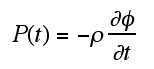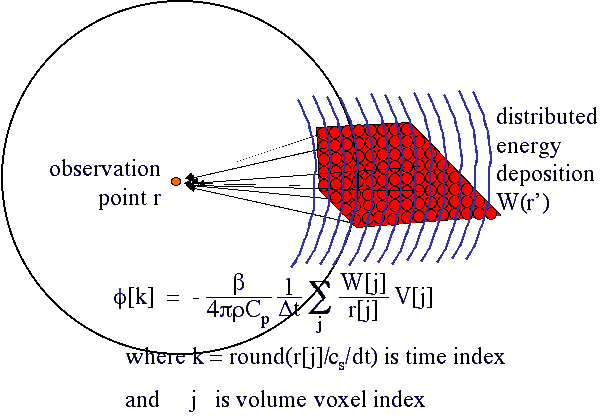# The basic idea

When energy is deposited in some region of tissue, the pressures generated by thermoelastic expansion propagate away from that region into the rest of the tissue. Consider the time-resolved pressure (P) arriving at an observation point, for example a detector, in response to an impulse of energy deposition (W) distributed over some region of tissue.

The pressure (P) [Pa] is related to a quantity called the velocity potential (φ) which is proportional to the energy deposition (W):where ρ is density [kg/m3], r is the position of observation, and t is time [s]. The following figure illustrates the problem and give the solution for calculating the velocity potential from a W(r).k indicates the kth time point, in steps of Δt = dr/cs . where cs = sound velocity (for water: 1480 m/s), and dr [m] is voxel size of simulation j indicates the jth voxel. φ is the velocity potential [m2/s]. V[j] is the jth voxel volume [m3]. r[j] is the jth voxel distance-from-observation-point [m]. W[j] is the jth voxel energy deposition [J/m3]. β is the thermal expansivity [strain/degree C], (for water: 2.29x10-4 strain/degree C). ρ is the density [kg/m3] (for water: 1000 kg/m3). Cp is the specific heat [J/(kg degreeC)], (for water: 4180 J/(kg degreeC)).

The velocity potential (φ) [m2/s] observed at time t is proportional to the sum of all energy deposition (W [J/m3]) at a distance r = cst, where cs is the speed of sound in the medium. An isotropic acoustic detector would detect the pressure arriving from a spherical shell of energy deposition, radius = r. An acoustic detector with a preferred direction of detection would detect from a spherical arc, radius = r.

The expression for calculating the velocity potential seen at an observation point at time t due to a field of energy deposition W(r), the red region described by voxels j = 1 to Nj, is shown in the above figure.